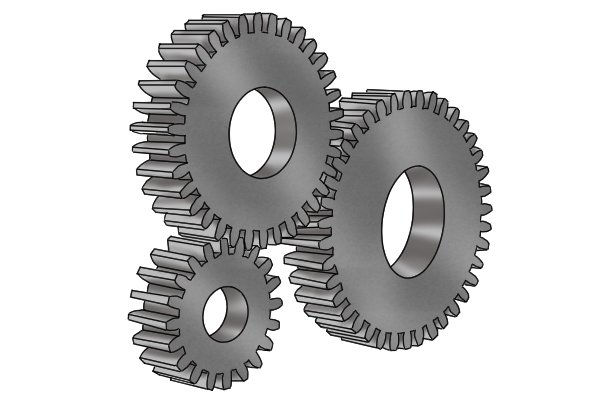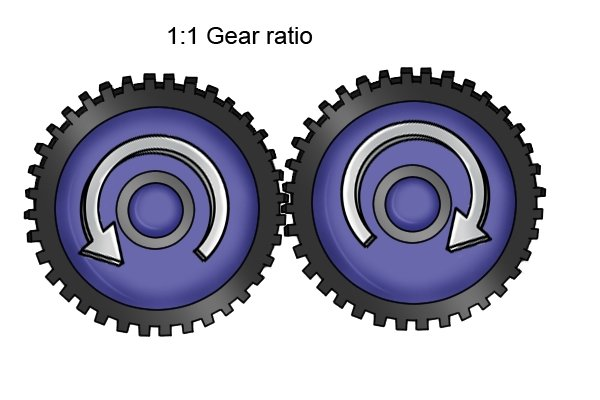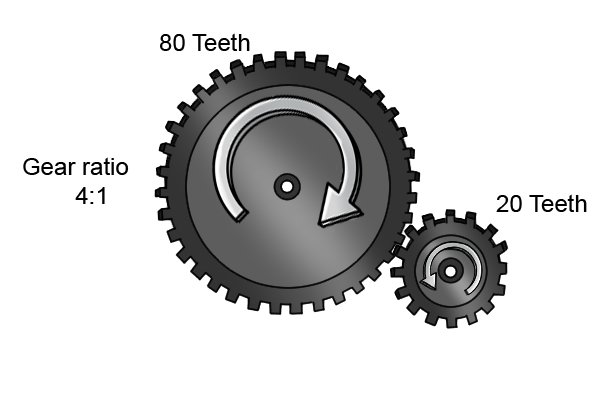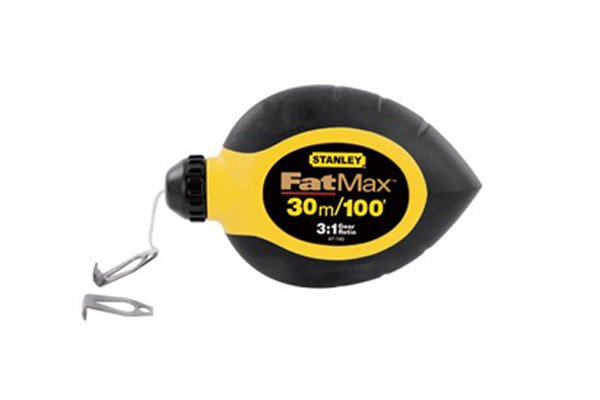# What is a chalk line’s gear ratio?

Shop for Chalk Lines

## Gear ratioGear ratio is a type of mechanical advantage; this means that it allows you to maximise a force while minimising effort (or vice versa). When a chalk line has a gear ratio higher than 1:1, it takes less effort to wind the string back in.### 1:1 gear ratio

A 1:1 gear ratio is when two cogs of the same size make a gear. As the rotational input force is equal to the output force, there is no mechanical advantage created. Put simply, for every one turn of the crank you will get one turn of the reel.### Can you work out your gear ratio?

Gear ratio can be worked out by counting the number of teeth that two cogs have and then dividing the number of teeth on the driving cog by the number the driven cog has. So, if the driving cog has 80 teeth and the driven cog has 20 teeth, you have a gear ratio of 4:1. This means that for every 1 rotation of input force you will get 4 rotations of output force.### Most common gear ratios

A lot of modern chalk lines have geared cranks which give you the benefit of being able to wind your string in faster and with less effort expended. The most common gear ratios are 1:1, 2:1, 3:1, 3.5:1, 4:1, and 5:1.### Which is best?

The higher the gear ratio, the quicker you can wind your string in e.g. a chalk line with 5:1 gear ratio will wind its string in five times faster than a chalk line with a 1:1 gear ratio.

Call Now Button